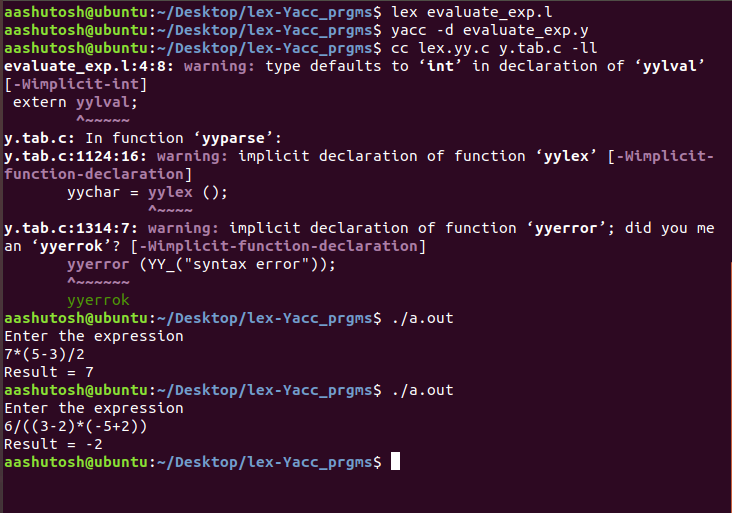Related Articles

# Yacc Program to evaluate a given arithmetic expression

• Difficulty Level : Hard
• Last Updated : 22 Mar, 2021

Prerequisite – Introduction to YACC
Problem: Write a YACC program to evaluate a given arithmetic expression consisting of ‘+’, ‘-‘, ‘*’, ‘/’ including brackets.
Examples:

```Input: 7*(5-3)/2
Output: 7

Input: 6/((3-2)*(-5+2))
Output: -2 ```

Lexical Analyzer Source Code:

## C

 `%{``    ``/* Definition section*/``    ``#include "y.tab.h"``    ``extern` `yylval;``}%` `%%``[0-9]+    {``              ``yylval = ``atoi``(yytext);``              ``return` `NUMBER;``            ``}` `[a-zA-Z]+    { ``return` `ID; }``[ \t]+         ;  ``/*For skipping whitespaces*/` `\n            { ``return` `0; }``.            { ``return` `yytext; }` `%%`

Parser Source Code:

## C

 `%{``    ``/* Definition section */``  ``#include ``%}` `%token NUMBER ID``// setting the precedence``// and associativity of operators``%left ``'+'` `'-'``%left ``'*'` `'/'` `/* Rule Section */``%%``E : T        {``                ``printf``(``"Result = %d\n"``, \$\$);``                ``return` `0;``            ``}` `T :``    ``T ``'+'` `T { \$\$ = \$1 + \$3; }``    ``| T ``'-'` `T { \$\$ = \$1 - \$3; }``    ``| T ``'*'` `T { \$\$ = \$1 * \$3; }``    ``| T ``'/'` `T { \$\$ = \$1 / \$3; }``    ``| ``'-'` `NUMBER { \$\$ = -\$2; }``    ``| ``'-'` `ID { \$\$ = -\$2; }``    ``| ``'('` `T ``')'` `{ \$\$ = \$2; }``    ``| NUMBER { \$\$ = \$1; }``    ``| ID { \$\$ = \$1; };``% %` `int` `main() {``    ``printf``(``"Enter the expression\n"``);``    ``yyparse();``}` `/* For printing error messages */``int` `yyerror(``char``* s) {``    ``printf``(``"\nExpression is invalid\n"``);``}`

Output:Notes:
Yacc programs are generally written in 2 files one for lex with .l extension(for tokenization and send the tokens to yacc) and another for yacc with .y extension (for grammar evaluation and result evaluation).
Steps for execution of Yacc program:

```yacc -d sample_yacc_program.y
lex sample_lex_program.l
cc lex.yy.c y.tab.c -ll
./a.out```

Attention reader! Don’t stop learning now. Get hold of all the important CS Theory concepts for SDE interviews with the CS Theory Course at a student-friendly price and become industry ready.

My Personal Notes arrow_drop_up